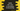# 5 different ways to sort a list in ascending/descending order in Kotlin# How to sort items of a list in Kotlin :

Sorting items of a list is one of the commonly faced step in software development. Not only in Kotlin, you will face it today or tomorrow in any programming language. Sorting items in ascending or descending order in a list is pretty easy for Kotlin.

Kotlin list comes with built-in methods for sorting items in ascending or descending orders. We can even sort custom objects easily in Kotlin. In this tutorial, we will learn how to sort items of a list in ascending and descending order.

## Sorting a simple integer list :

To sort a list in Kotlin, Collection class provides one method called sorted(). The syntax of the method is as below :

``public fun > Iterable.sorted(): List``

This method returns one new list containing all elements sorted in natural order.

Let’s try to implement it with an example :

``````fun main(args: Array) {
val numsList = listOf(1,5,2,88,33,56,23,10)

val sortAsc = numsList.sorted()
println(sortAsc)
}``````

If you run the above code, it will print the below output :

``[1, 2, 5, 10, 23, 33, 56, 88]``

As you can see that we have created the list with random numbers , but it was sorted after we have called the sort() method.

## Sort in descending order :

Similar as like ascending order sorting,we also have one built-in method for sorting a list in descending order : sortedDescending. The syntax of this method is :

``public fun > Iterable.sortedDescending(): List ``

It will return a list of elements sorted in descending order according to their natural sort order. Let’s modify the above example as below :

``````fun main(args: Array) {
val numsList = listOf(1,5,2,88,33,56,23,10)

val sortAsc = numsList.sortedDescending()
println(sortAsc)
}``````

It will print the below output :

``[88, 56, 33, 23, 10, 5, 2, 1]``

As you can see that sortedDescending() has reversed the list as per their values.

## Reverse a list :

Similar to sorting, we can also reverse a list easily in Kotlin. reversed() method is used to reverse a list. The syntax is as below :

``public fun  Iterable.reversed(): List``

It will reverse the list and return one new list with the elements in reversed order.

``````fun main(args: Array) {
val numsList = listOf(1,5,2,88,33,56,23,10)

val sortAsc = numsList.reversed()
println(sortAsc)
}``````

Output :

``[10, 23, 56, 33, 88, 2, 5, 1]``

## Sort a list of custom objects by its property :

We can also sort a list of custom objects easily in Kotlin.

• For ascending sorting , sortedBy() is used .
• For descending sorting , sortedByDescending() is used.

We need to pass one selector function for both of these methods and based on this function, the list is sorted in ascending or descending order. Let’s take a look at the program how to use these methods :

``````fun main(args: Array) {
val students = listOf(Student("Alex", 54), Student("Rob", 34),
Student("Chandler", 11), Student("Bryan", 90))

println("Sorting by ascending : ")
val sortedStudents = students.sortedBy { student -> student.name }
sortedStudents.forEach { s -> println(s.name) }

println("Sorting by descending : ")
val dSortedStudents = sortedStudents.sortedByDescending { student -> student.name }
dSortedStudents.forEach { s -> println(s.name) }
}

class Student(val name: String,marks : Int)``````

It will print the below output :

``````Sorting by ascending :
Alex
Bryan
Chandler
Rob
Sorting by descending :
Rob
Chandler
Bryan
Alex``````

## Sort using a comparator :

Instead of doing the ascending or descending sorting, we can also sort a list using a comparator. sortedWith() method is used to sort a list using a comparator. The comparator is passed as an argument to the function.

``````fun main(args: Array) {
val students = listOf(Student("Alex", 54), Student("Rob", 34),
Student("Chandler", 11), Student("Bryan", 90))

println("Sorting using a comparator : ")
val sortedStudents = students.sortedWith (Comparator { student1, student2 -> student1.name.length - student2.name.length })
sortedStudents.forEach { s -> println(s.name) }
}

class Student(val name: String,marks : Int)``````

Output :

``````Sorting using a comparator :
Rob
Alex
Bryan
Chandler``````

As you can see that we have sorted the list as per the length of the name string. You can create one custom Comparator class and modify the comparision as per your requirement.

### Conclusion :

In this tutorial, we have learn how to sort a list in ascending/descending order, how to reverse a list, how to sort a list of custom objects and how to sort a list using custom comparator. Go through the examples listed above and drop one comment below if you have any queries.

You might also like :